# Class 11 Maths NCERT Solutions for Chapter 16 Probability Exercise 16.2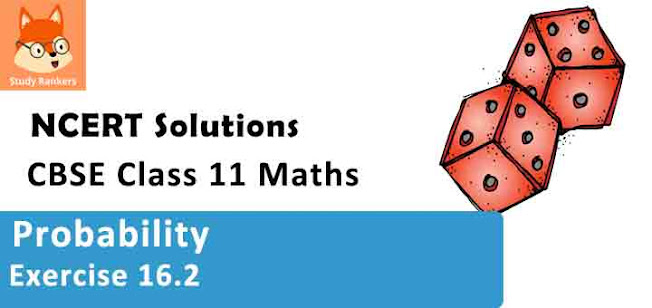### Statistics Exercise 16.2 Solutions

1. A die is rolled. Let E be the event “die shows 4” and F be the event “die shows even number”. Are E and F mutually exclusive?

Solution

When a die is rolled, the sample space is given by
S = {1, 2, 3, 4, 5, 6}
Accordingly, E = {4} and F = {2, 4, 6}
It is observed that E ∩ F = {4} ≠ Φ
Therefore, E and F are not mutually exclusive events.

2. A die is thrown. Describe the following events:
(i) A: a number less than 7
(ii) B: a number greater than 7
(iii) C: a multiple of 3
(iv) D: a number less than 4
(v) E: an even number greater than 4 (vi) F: a number not less than 3
Also find A ∪ B, A ∩ B, B ∪ C, E ∩ F, D ∩ E, A – C, D – E, E Ç F', F'

Solution

When a die is thrown, the sample space is given by S = {1, 2, 3, 4, 5, 6}.
Accordingly:
(i) A = {1, 2, 3, 4, 5, 6}
(ii) B = Φ
(iii) C = {3, 6}
(iv) D = {1, 2, 3}
(v) E = {6}
(vi) F = {3, 4, 5, 6}
A ∪ B = {1, 2, 3, 4, 5, 6}, A ∩ B = Φ
B ∪ C = {3, 6}, E ∩ F = {6}
D ∩ E =Φ, A – C = {1, 2, 4, 5}
D – E = {1, 2, 3}, F' = {1, 2}, E ∩ F' = ∅

3. An experiment involves rolling a pair of dice and recording the numbers that come up. Describe the following events:
A: the sum is greater than 8, B: 2 occurs on either die
C: The sum is at least 7 and a multiple of 3.
Which pairs of these events are mutually exclusive?

Solution

When a pair of dice is rolled, the sample space is given by
S = {(x, y) : x , y = 1, 2, 3, 4, 5, 6 }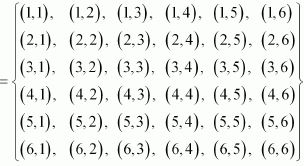Accordingly,
A = {(3, 6) , (4,5), (4,6),(5,4),(5,5),(5,6),(6,3),(6,4),(6,5),(6,6)}
B = { (2,1), (2,2), (2,3),(2,4),(2,5),(2,6),(1,2),(3,2),(4,2),(5,2),(6,2)}
C = {(3,6),(4,5),(5,4),(6,3),(6,6)}
It is observed that
A ∩ B =Φ
B ∩ C =Φ
C∩A = {(3,6),(4,5),(5,4),(6,3),(6,6)} = Φ
Hence, events A and B and events B and C are mutually exclusive.

4. Three coins are tossed once. Let A denote the event "three heads show", B denote the event "two heads and one tail show". C denote the event "three tails show" and D denote the event "a head shows on the first coin". Which events are
(i) mutually exclusive?
(ii) simple?
(iii) compound?

Solution

When three coins are tossed, the sample space is given by
S = {HHH, HHT, HTH, HTT, THH, THT, TTH, TTT}
Accordingly,
A = {HHH}
B = {HHT, HTH, THH}
C = {TTT}
D = {HHH, HHT, HTH, HTT}
We now observe that
A ∩ B =Φ, A ∩ C =Φ, A ∩ D = {HHH} ≠ Φ
B ∩ C =Φ, B ∩ D = {HHT, {HTH} ≠ Φ
C ∩ D = Φ

(i) Event A and B; event A and C; event B and C; and event C and D are all mutually exclusive.

(ii) If an event has only one sample point of a sample space, it is called a simple event. Thus, A and
C are simple events.

(iii) If an event has more than one sample point of a sample space, it is called a compound event.
Thus, B and D are compound events.

5. Three coins are tossed. Describe
(i) Two events which are mutually exclusive.
(ii) Three events which are mutually exclusive and exhaustive.
(iii) Two events, which are not mutually exclusive.
(iv) Two events which are mutually exclusive but not exhaustive.
(v) Three events which are mutually exclusive but not exhaustive.

Solution

When three coins are tossed, the sample space is given by
S = {HHH, HHT, HTH, HTT, THH, THT, TTH, TTT}

(i) Two events that are mutually exclusive can be
A: getting no heads and B: getting no tails
This is because sets A = {TTT} and B = {HHH} are disjoint.

(ii) Three events that are mutually exclusive and exhaustive can be
C: getting at least two heads
i.e.,
A = {TTT}
B = {HTT, THT, TTH}
C = {HHH, HHT, HTH, THH}
This is because A ∩ B = B ∩ C = C ∩ A = Φand A ∪ B ∪ C = S

(iii) Two events that are not mutually exclusive can be
B: getting at least 2 heads
i.e.,
A = {HHH}
B = {HHH, HHT, HTH, THH}
This is because A ∩ B = {HHH} ≠ Φ

(iv) Two events which are mutually exclusive but not exhaustive can be
B: getting exactly one tail
That is
A = {HTT, THT, TTH}
B = {HHT, HTH, THH}
It is because, A ∩ B =Φ, but A ∪ B ≠ S

(v) Three events that are mutually exclusive but not exhaustive can be
B: getting one head and two tails
C: getting one tail and two heads
i.e.,
A = {HHH}
B = {HTT, THT, TTH}
C = {HHT, HTH, THH}
This is because A ∩ B = B ∩ C = C ∩ A = Φ, but A ∪ B ∪ C ≠ S

6. Two dice are thrown. The events A, B and C are as follows:
A: getting an even number on the first die.
B: getting an odd number on the first die.
C: getting the sum of the numbers on the dice ≤ 5
Describe the events
(i) A' (ii) not B (iii) A or B
(iv) A and B (v) A but not C (vi) B or C
(vii) B and C (viii) A ∩ B'∩C

Solution

When two dice are thrown, the sample space is given by
S = {(x, y) : x , y = 1 ,2,3,4,5,6,}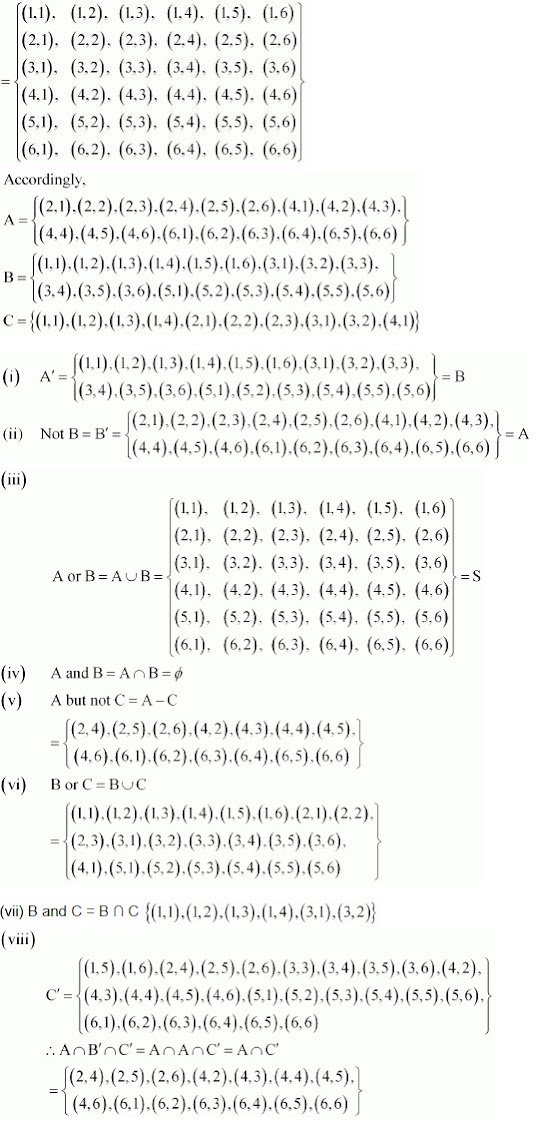7. Two dice are thrown. The events A, B and C are as follows:
A: getting an even number on the first die.
B: getting an odd number on the first die.
C: getting the sum of the numbers on the dice ≤ 5
(i) A and B are mutually exclusive
(ii) A and B are mutually exclusive and exhaustive
(iii) A = B'
(iv) A and C are mutually exclusive
(v) A and B' are mutually exclusive
(vi) A', B', C are mutually exclusive and exhaustive.

Solution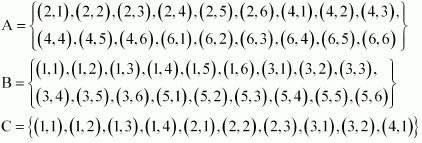(i) It is observed that A ∩ B = Φ
∴ A and B are mutually exclusive.
Thus, the given statement is true.

(ii) It is observed that A ∩ B = Φ and A ∪ B = S
∴ A and B are mutually exclusive and exhaustive.
Thus, the given statement is true.

(iii) It is observed that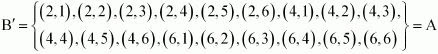Thus, the given statement is true.

(iv) It is observed that A ∩ C = {(2, 1), (2, 2), (2, 3), (4, 1)} ≠Φ
∴A and C are not mutually exclusive.
Thus, the given statement is false.

(v) A ∩ B' = A ∩A = A
∴ A ∩ B' ≠ Φ
∴ A and B' are not mutually exclusive.
Thus, the given statement is false.

(vi) It is observed that; A' ∪ B'  ∪ C = S;
However, B' ∩ C = {(2, 1), (2,2), (2, 3),(4, 1)} ≠ Φ
Therefore, events A' , B' are C not mutually exclusive and exhaustive.
Thus, the given statement is false.